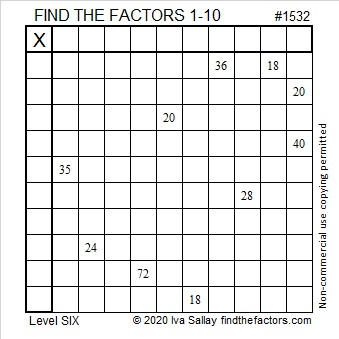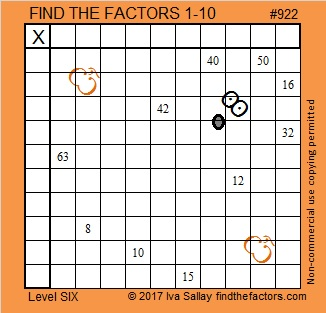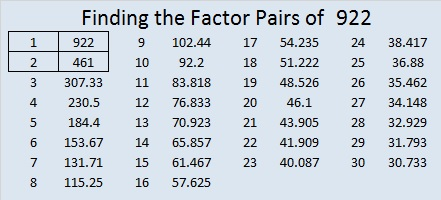# 1532 Don’t Let This Puzzle Spook You!

### Today’s Puzzle:

If this little ghost prowls your neighborhood this Halloween, don’t let it spook you. Sure, it is a level 6 puzzle, but if you stick to using logic from start to finish, you’ll know the most about this ghost!Here’s the same puzzle minus the embellishments:If you know what a normal distribution is, then you should enjoy this statistics joke:

### Factors of 1532:

• 1532 is a composite number.
• Prime factorization: 1532 = 2 × 2 × 383, which can be written 1532 = 2² × 383.
• 1532 has at least one exponent greater than 1 in its prime factorization so √1532 can be simplified. Taking the factor pair from the factor pair table below with the largest square number factor, we get √1532 = (√4)(√383) = 2√383.
• The exponents in the prime factorization are 2 and 1. Adding one to each exponent and multiplying we get (2 + 1)(1 + 1) = 3 × 2 = 6. Therefore 1532 has exactly 6 factors.
• The factors of 1532 are outlined with their factor pair partners in the graphic below.### More about the Number 1532:

1532 = 2 × 383 × 2. That factorization looks the same frontwards or backward.

1532 can be written as the difference of two squares:
384² – 382² = 1532.

# 922 Boo!

Have you ever cut holes in a sheet, put it over your head, and jumped out in front of people as you hollered, “Boo!”? Today’s puzzle is made to look like a ghost. It’s a level 6, but don’t let that spook you! Attack the puzzle using logic, and after you solve it, you can claim to be a ghost-buster!Print the puzzles or type the solution on this excel file: 10-factors-914-922

I think my ghost is cute, maybe not as cute as any that might knock on your door on Halloween, but still quite cute.

When 922 floats around in a different base, you may think you’re seeing an apparition:

922 becomes 1234 in BASE 9 because 1(9³) + 2(9²) + 3(9¹) + 4(9º) = 922.
922 becomes palindrome 262 in BASE 20

922 is also the sum of the 18 prime numbers from 17 to 89.

922 = 29² + 9², so 922 is the hypotenuse of a Pythagorean triple:
522-760-922, which is the same as 2(29)(9), 29² – 9², 29² + 9².
That Pythagorean triple is also 2 times (261-380-461).

• 922 is a composite number.
• Prime factorization: 922 = 2 × 461
• The exponents in the prime factorization are 1 and 1. Adding one to each and multiplying we get (1 + 1)(1 + 1) = 2 × 2 = 4. Therefore 922 has exactly 4 factors.
• Factors of 922: 1, 2, 461, 922
• Factor pairs: 922 = 1 × 922 or 2 × 461
• 922 has no square factors that allow its square root to be simplified. √922 ≈ 30.3644529